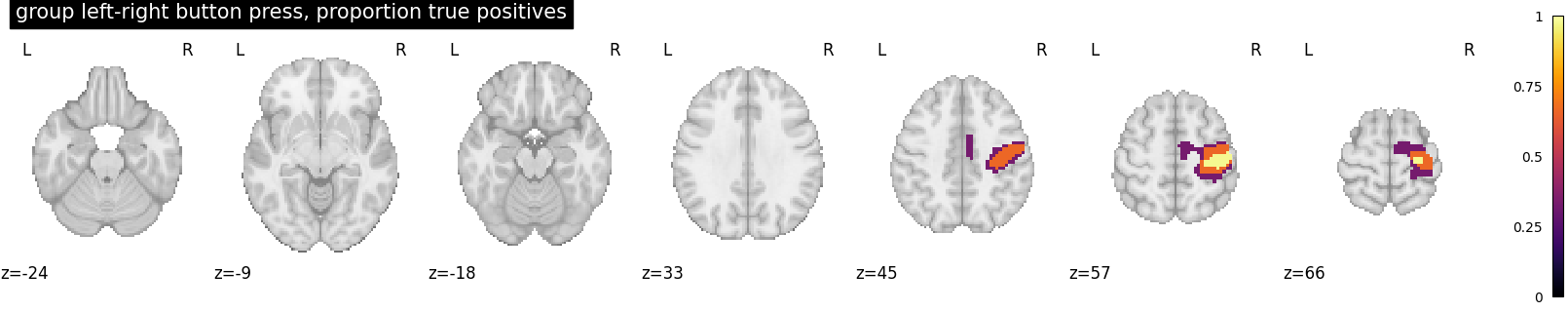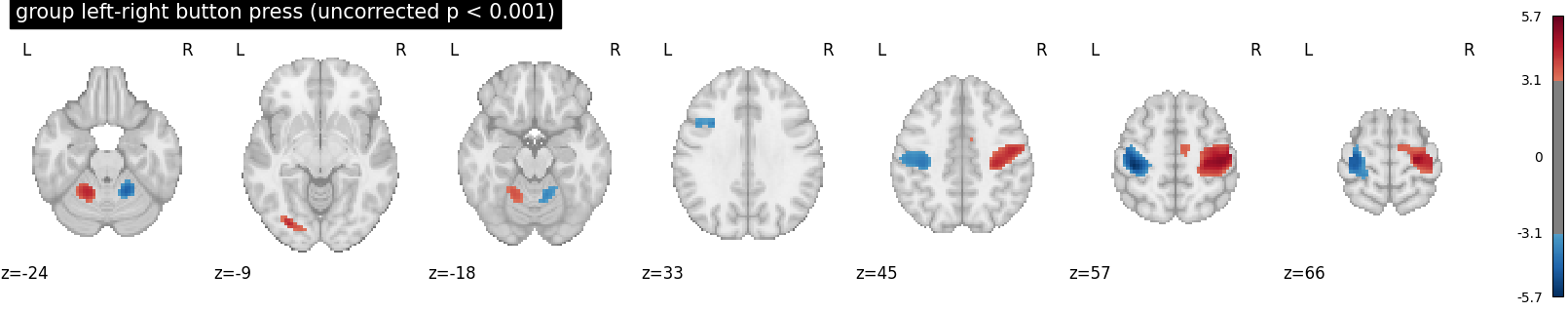# Second-level fMRI model: true positive proportion in clusters#

This script showcases the so-called “All resolution inference” procedure, in which the proportion of true discoveries in arbitrary clusters is estimated. The clusters can be defined from the input image, i.e. in a circular way, as the error control accounts for arbitrary cluster selection.

Rosenblatt JD, Finos L, Weeda WD, Solari A, Goeman JJ. All-Resolutions Inference for brain imaging. Neuroimage. 2018 Nov 1;181:786-796. doi: 10.1016/j.neuroimage.2018.07.060

## Fetch dataset#

We download a list of left vs right button press contrasts from a localizer dataset. Note that we fetch individual t-maps that represent the BOLD activity estimate divided by the uncertainty about this estimate.

```from nilearn.datasets import fetch_localizer_contrasts

n_subjects = 16
data = fetch_localizer_contrasts(
["left vs right button press"],
n_subjects,
get_tmaps=True,
legacy_format=False,
)
```

## Estimate second level model#

We define the input maps and the design matrix for the second level model and fit it.

```import pandas as pd

second_level_input = data["cmaps"]
design_matrix = pd.DataFrame(
 * len(second_level_input), columns=["intercept"]
)
```

Model specification and fit

```from nilearn.glm.second_level import SecondLevelModel

second_level_model = SecondLevelModel(smoothing_fwhm=8.0)
second_level_model = second_level_model.fit(
second_level_input, design_matrix=design_matrix
)
```

To estimate the contrast is very simple. We can just provide the column name of the design matrix.

```z_map = second_level_model.compute_contrast(output_type="z_score")
```

We threshold the second level contrast at uncorrected p < 0.001 and plot

```from scipy.stats import norm

p_val = 0.001
p001_uncorrected = norm.isf(p_val)

from nilearn.glm import cluster_level_inference

proportion_true_discoveries_img = cluster_level_inference(
z_map, threshold=[3, 4, 5], alpha=0.05
)

from nilearn import plotting

plotting.plot_stat_map(
proportion_true_discoveries_img,
threshold=0.0,
display_mode="z",
vmax=1,
colorbar=True,
title="group left-right button press, proportion true positives",
)

plotting.plot_stat_map(
z_map,
threshold=p001_uncorrected,
colorbar=True,
display_mode="z",
title="group left-right button press (uncorrected p < 0.001)",
)

plotting.show()
```
••Total running time of the script: ( 0 minutes 13.871 seconds)

Estimated memory usage: 9 MB

Gallery generated by Sphinx-Gallery